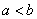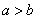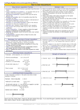How to Learn in 24 Hours?The Rapid Learning Movie

 Need Help? M-F: 9am-5pm(PST): Toll-Free: (877) RAPID-10 US Direct: (714) 692-2900 Int'l: 001-714-692-2900 24/7 Online Technical Support: The Rapid Support Center Secure Online Order:Need Proof? Testimonials by Our Users

 Rapid Learning Courses: MCAT in 24 Hours (2015-16) USMLE in 24 Hours (Boards) Chemistry in 24 Hours Biology in 24 Hours Physics in 24 Hours Mathematics in 24 Hours Psychology in 24 Hours SAT in 24 Hours ACT in 24 Hours AP in 24 Hours CLEP in 24 Hours DAT in 24 Hours (Dental) OAT in 24 Hours (Optometry) PCAT in 24 Hours (Pharmacy) Nursing Entrance Exams Certification in 24 Hours eBook - Survival Kits Audiobooks (MP3)

 Tell-A-Friend: Have friends taking science and math courses too? Tell them about our rapid learning system.Home »  Mathematics »  College Algebra

Linear Equations

 Topic Review on "Title": Equations:  Equations are statements where two quantities have the same value. Golden Rule: Whenever the same arithmetic operations are performed on both sides the equation will remain true. Domain:  The domain is the set of all possible values that the variables can have. Solution Set: The solution set is the collection of all roots of an equation. Equivalent Equations: Equivalent equations are equations that have the same solution set. Proportions: Proportions are equations that state that two fractions are equal. Identity: An identity is an equation with one variable that has no solution. Inequality Signs: The inequality signs are read from left to right and are defined as:a is less than ba is less than or equal to ba is greater than or equal to ba is greater than or equal to b

Rapid Study Kit for "Title":
 Flash Movie Flash Game Flash Card Core Concept Tutorial Problem Solving Drill Review Cheat Sheet"Title" Tutorial Summary : This tutorial describes linear equations and their use in application problems. Arithmetic operations techniques are used to show the importance of solving linear equations for unknown variables. Inverse operations are mentioned to emphasize their use in solving linear equations. Key concepts such as proportions, linear inequalities are shown with the use of examples and strategies. A correlation of linear equations with linear inequalities is described with the use of solving techniques. Finally, an example showing how you solve multiple inequalities is described in this tutorial.

 Tutorial Features: Specific Tutorial Features: • Step by step examples showing solving linear equations, solving proportions and linear inequalities are shown in this tutorial. • Examples displaying how to solve linear inequalities and how the solutions are represented using interval notation are displayed in this tutorial. Series Features: • Concept map showing inter-connections of new concepts in this tutorial and those previously introduced. • Definition slides introduce terms as they are needed. • Visual representation of concepts • Animated examples—worked out step by step • A concise summary is given at the conclusion of the tutorial.

 "Title" Topic List: Definition of Linear Equations Golden Rule Solving a Linear Equation Strategies to solve a linear equation Identity Linear Inequalities Definition of Linear Inequalities Linear Inequalities Signs Solving linear inequalities Interval Notation

See all 24 lessons in College Algebra, including concept tutorials, problem drills and cheat sheets:
Teach Yourself College Algebra Visually in 24 Hours